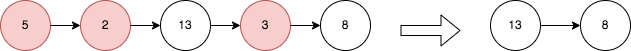##### Welcome to Subscribe On Youtube

Formatted question description: https://leetcode.ca/all/2487.html

# 2487. Remove Nodes From Linked List

• Difficulty: Medium.
• Related Topics: Linked List, Stack, Recursion, Monotonic Stack.
• Similar Questions: Reverse Linked List, Delete Node in a Linked List, Next Greater Element I.

## Problem

You are given the head of a linked list.

Remove every node which has a node with a strictly greater value anywhere to the right side of it.

Return the **head of the modified linked list.**

Example 1:Input: head = [5,2,13,3,8]
Output: [13,8]
Explanation: The nodes that should be removed are 5, 2 and 3.
- Node 13 is to the right of node 5.
- Node 13 is to the right of node 2.
- Node 8 is to the right of node 3.


Example 2:

Input: head = [1,1,1,1]
Output: [1,1,1,1]
Explanation: Every node has value 1, so no nodes are removed.


Constraints:

• The number of the nodes in the given list is in the range [1, 105].

• 1 <= Node.val <= 105

## Solution (Java, C++, Python)

• /**
* public class ListNode {
*     int val;
*     ListNode next;
*     ListNode() {}
*     ListNode(int val) { this.val = val; }
*     ListNode(int val, ListNode next) { this.val = val; this.next = next; }
* }
*/
class Solution {
List<Integer> nums = new ArrayList<>();
}
Deque<Integer> stk = new ArrayDeque<>();
for (int v : nums) {
while (!stk.isEmpty() && stk.peek() < v) {
stk.pop();
}
stk.push(v);
}
ListNode dummy = new ListNode();
while (!stk.isEmpty()) {
}
return dummy.next;
}
}

• /**
* struct ListNode {
*     int val;
*     ListNode *next;
*     ListNode() : val(0), next(nullptr) {}
*     ListNode(int x) : val(x), next(nullptr) {}
*     ListNode(int x, ListNode *next) : val(x), next(next) {}
* };
*/
class Solution {
public:
vector<int> nums;
}
vector<int> stk;
for (int v : nums) {
while (!stk.empty() && stk.back() < v) {
stk.pop_back();
}
stk.push_back(v);
}
ListNode* dummy = new ListNode();
for (int v : stk) {
}
return dummy->next;
}
};

• # Definition for singly-linked list.
# class ListNode:
#     def __init__(self, val=0, next=None):
#         self.val = val
#         self.next = next
class Solution:
def removeNodes(self, head: Optional[ListNode]) -> Optional[ListNode]:
nums = []
stk = []
for v in nums:
while stk and stk[-1] < v:
stk.pop()
stk.append(v)
dummy = ListNode()
for v in stk:
return dummy.next


• /**
* type ListNode struct {
*     Val int
*     Next *ListNode
* }
*/
nums := []int{}
}
stk := []int{}
for _, v := range nums {
for len(stk) > 0 && stk[len(stk)-1] < v {
stk = stk[:len(stk)-1]
}
stk = append(stk, v)
}
dummy := &ListNode{}
for _, v := range stk {
}
return dummy.Next
}


Explain:

nope.

Complexity:

• Time complexity : O(n).
• Space complexity : O(n).